CIS 5511. Programming Techniques

Probabilistic Analysis

### 1. Average cost

For a given problem S, if each instance of a given size si, i = 1..n has a cost ci, then the best case and the worst case cost are Minimum(ci, i = 1..n) and Maximum(ci, i = 1..n), respectively, while the average case cost is ∑pici, where i = 1 .. n, and pi is the probability for the instance si to be taken as the input of the algorithm. In the current context, the probability pi is defined by Lim[t → ∞] (ti / t), where t is the total number of times the algorithm is used, and ti is the number for instance si to show up in the process, i.e., its occurrence frequency.

As a special situation, when all instances have the same probability to show up, we have pi = p. Since ∑[i = 1..n] pi = 1, then ∑[i = 1..n] pi = np = 1, so p = 1/n, and the average cost ∑[i = 1..n] pici = (∑[i = 1..n] ci) / n.

To get the probability values is not always easy. Usually, we have to depend on some assumptions reflecting our current knowledge about the problem. As the situation changes, we may need to redo the analysis.

### 2. Example: the hiring problem

For example, the algorithm given in the textbook hires a new office assistant right away as far as the new candidate is the best so far: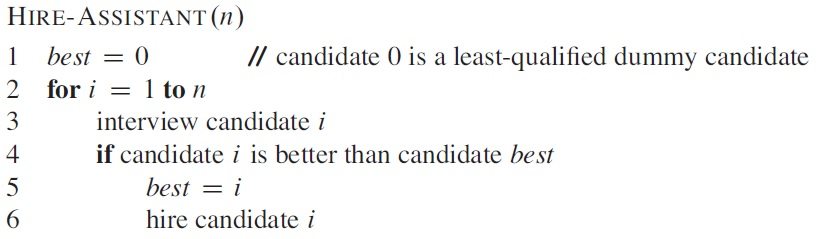There are some unusual properties in this example:

• As an application problem, the steps are not fully formalized.
• The input does not have to be available at the same time (at the very beginning), and the output does not have to be available at the same time (at the very end), neither.
• The goal is to analyze the cost of the procedure, reflected by line 3 and 6, not the running time of the algorithm.
Even so, this algorithm can be analyzed as before. Assuming the cost of interviewing and hiring is ci and ch, respectively, then the cost of the above algorithm is O(n ci + m ch), where m is the number of hiring. Since the interview cost n ci remains unchanged, our analysis will focus on the hiring cost m ch.

Obviously, for this problem the best case happens when the best candidate comes first, and the worst case happens when every candidate is better than the previous ones.

To calculate the average hiring cost, we assume that the candidates come in random order, meaning that each possible order is equally likely. Please notice that such an assumption is different from a "complete ignorance" or "pure arbitrary" assumption --- we don't know which input the algorithm will meet each time, but we do assume that in the long run all possibilities will happen equally often. In general, it is not always valid to treat an uncertain variable as a random variable.

To calculate the probability for line 5 and 6 to be executed in the above algorithm, we can see that candidate i is hired, in line 5, exactly when he/she is better than each of candidates 1 through i-1. Since any one of the first i candidates is equally likely to be the best so far, candidate i has probability of 1/i to be hired. The expected number of hires is ∑[i = 1..n] 1/i = ln n + O(1), which is O(ln n) (Page 1147, A.7).

### 3. Randomized algorithm

As mentioned before, we cannot always assume that the input of an algorithm contains random variables with known probabilistic distributions. For example, in the Hire-Assistant algorithm, when the coming order of candidate cannot be assumed to be random, the above average cost estimation is no longer valid.

One way to avoid this situation is to actually impose a probabilistic distribution onto the input, so as to turn it into a random variable. The common way to do so is to use a pseudo-random-number generator to rearrange the input. In this way, no matter how the input is presented to the system, the average computational cost is guaranteed to fit the probabilistic analysis. Such an algorithm is called "randomized". Please note that this solution assumes that all candidates are all available for processing at the very beginning.

For such a randomized algorithm, no particular input always has the best-case, or the worst-case, of time cost.

Many randomized algorithms randomize the input by permuting the given input array A. One common method for doing so is to assign each element in the array A[i] a random priority P[i], and then sort the elements of A according to their priorities: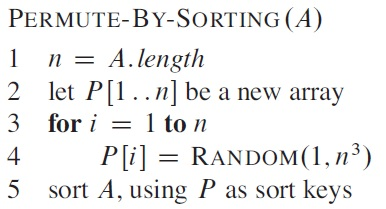The range [1, n3] is chosen to reduce duplicates in the random numbers generated.

A better method for generating a random permutation is to permute the given array in place. In iteration i, the element A[i] is chosen randomly from subarray A[i..n], then remain unchanged.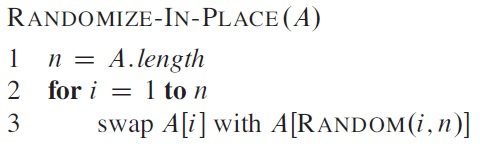It can be proven that both algorithms generate random permutation as desired.

### 4. Example: the on-line hiring problem

As a variant of the hiring problem, suppose now we can only hire once immediately after an interview (so we may not interview all the candidates). For this new problem, what is the trade-off between minimizing the amount of interviewing and maximizing the quality of the candidate hired?

Let's assume that after an interview we can assign the candidate a score, and no two candidates have the same score. One solution is: first select a positive integer k < n, interview but reject the first k candidates, then hire the first candidate thereafter who has a higher score than the best of the first k (which is also the best of all the preceding) candidates. If no such one can be found, hire the last one.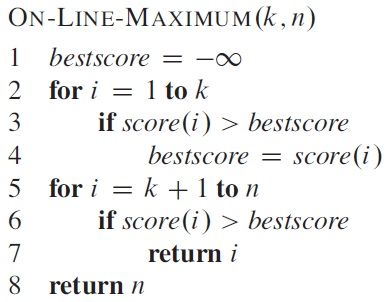To decide the best choice of k that gives the highest probability for the best candidate to be hired, Pr{S}. We divide this event into the events where the hiring happens when the best candidate is at position i: Pr{S} = ∑[i = 1..n] Pr{Si}. Since the first k candidates are all rejected, it is actually ∑[i = k+1..n] Pr{Si}.

The event Si happens if and only if (1) the best candidate is at position i, and (2) nobody before it is better than the best of the first k candidates. The first value is 1/n, and the second one is k/(i-1). In summary,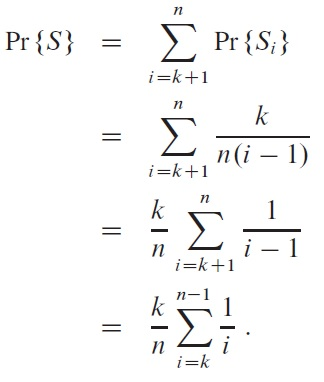Pr{S} is maximized when k = n/e, with the value 1/e, about 37%.

Please note that the above Pr{S} is just one of several possible measurements that can be used to select k.